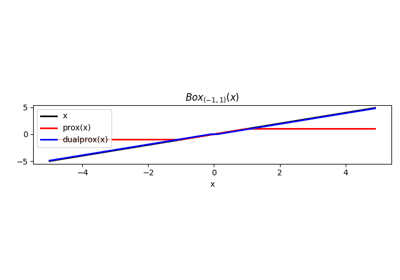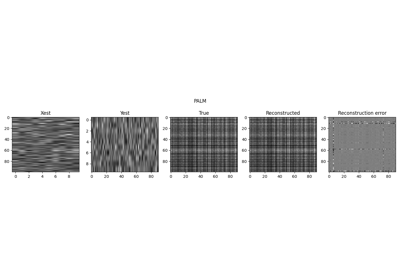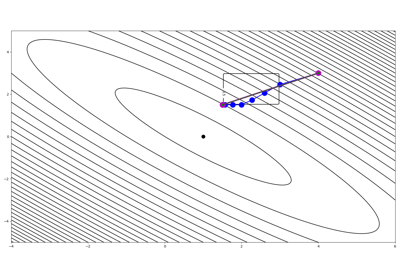# pyproximal.Box#

class pyproximal.Box(lower=- inf, upper=inf)[source]#

Box proximal operator.

Proximal operator of a Box: $$\operatorname{Box}_{[l, u]} = \{ x: l \leq x\leq u \}$$.

Parameters
lowerfloat or np.ndarray, optional

Lower bound

upperfloat or np.ndarray, optional

Upper bound

Notes

As the Box is an indicator function, the proximal operator corresponds to its orthogonal projection (see pyproximal.projection.BoxProj for details.

Methods

 __init__([lower, upper]) affine_addition(v) Affine addition chain(g) Chain grad(x) Compute gradient postcomposition(sigma) Postcomposition precomposition(a, b) Precomposition prox(**kwargs) proxdual(**kwargs)

## Examples using pyproximal.Box#Norms

NormsLow-Rank completion via Matrix factorization

Low-Rank completion via Matrix factorizationNonlinear inversion with box constraints

Nonlinear inversion with box constraints